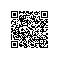# PHP防止数据库数字SQL注入攻击

// 防止SQL注入
if (empty($id) || !is_numeric($id))
{
die("警告：这个是非法数字！");
}
// 连接数据库
$conn=mysql_connect($Db_Server,$Db_User,$Db_Pwd);
// 判断连接是否成功
if (!$conn) { die("不能连接数据库，错误是: " . mysql_error()); } // 数据库输出编码，应该与你的数据库编码保持一致。建议用UTF-8国际标准编码 mysql_query("set names 'utf8'"); // 打开数据库$db_selected = mysql_select_db($Db_Name,$conn);
// 判断打开是否成功
if (!$db_selected) { die ("不能打开数据库，错误是: " . mysql_error()); } // SQL语句$sql ="select * from demo where id=".$id; // 查询SQL语句$result = mysql_query($sql,$conn);
// 判断是否有数据
if (!$result) { die("查询失败: " . mysql_error()); } // 循环读取数据 while($row = mysql_fetch_array($result)) { // 读数据 echo$row['content'];
}
// 使用完毕关闭数据库连接
mysql_close();
?>使用钉钉扫一扫加入圈子
+ 订阅